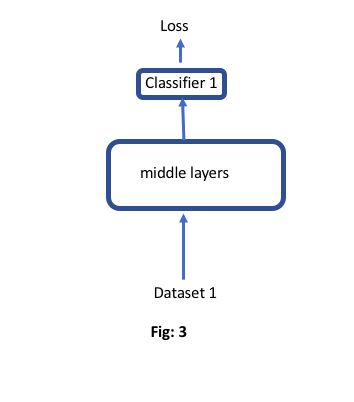Let’s say following multi-task learning setting like Fig:1, where we have two related datasets (dataset 1 and dataset 2). For each iteration creat a batch of 64 (32+32) that goes through some layers. Each dataset has different classifiers. Next to those common middle layers, there are two classifiers for each dataset. Both losses affect on middle layers.

Now, let’s say another experimental situation (fig: 2), where I don’t calculate loss 2 and its gradients, But both datasets go through the middle layers. Here, I only calculate loss 1 and it optimizes using this loss.

My question is, should not the gradient calculation and optimization be the same of if I don’t use the second dataset at all like fig:3? Because, I think, the computation graph of loss 1 (fig:2) does not contain any information about the second dataset.

But, this is not happening in my case. Fig:3 model optimizes much better and give better performance than fig: 2Hi,

The major differences I can think of are:

• The batch size might be half of what you expect?
• Layers like batchnorm will behave differently as they won’t see samples from the second dataset.
• For calculating loss 1, the batch size is 32 for both models.

• There is no batch norm layer. The middle layer actually is a CNN layer

If there is only a CNN layer, then all will be the same from pytorch point of view.
Most likely a different config in your code between the two no?

So, I did some debugging and inspections. I fixed the input and weights of the models.
Based on some experiments, I think that there is an effect of dataset 2.
Here are the experiments I did:

1. I found that problem (the output dissimilarities) is not in optimization, the problem occurs from the very first prediction (before even first call of `.backward()`). The predictions of first batch of dataset 1 is different in figure 2 and figure 3.

2. At fig 2 model, I stop passing the dataset 2 through CNN and now the prediction is exactly the same as the model of fig 3. This already showing the effect of dataset 2. But I did one further experiment for more confidence.

3. If I change the examples of dataset 2 (by changing random seed) for creating one batch (examples of dataset 1 + dataset 2 ) for model of fig:2, the prediction for the example of dataset 1 also change each time. If I fix the dataset 2 examples, the prediction of dataset 1 examples is always the same.

Now, the only thing I can think of is changing due to the addition of dataset 2, is the padding. When everything is fixed (weights and examples), the addition of another dataset creates different padding (The padded dataset 1 input will be different between fig 2 and fig 3). I used the default zero padding and the data is natural language not image. Pdding is used because sentences and documents that are of variable sizes.

Do you think, this might be the issue?

Well if there is only a single conv layer in the middle, it won’t change anything.
So it will depend on what other code you have the behaves differently in both cases indeed.